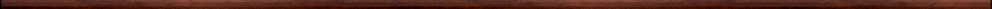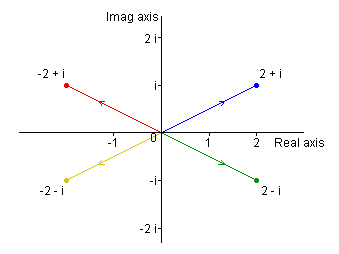Maple worksheets on complex numbersVectors, matrices and complex numbers:

They are all compatible with Classic Worksheet Maple 10.An Introduction to complex numbers - complex.mws

• Solving quadratic equations and the imaginary unit.
• Argand diagrams, the modulus and conjugate of a complex number.
• Arithmetic operations on complex numbers.
• A note on programming complex arithmetic.

The polar form for complex numbers - polar.mws

• The argument of a complex number.
• The polar form of a complex number.
• Multiplication and division of complex numbers in polar form.

Plotting complex solutions of equations - plotcomplex.mws

• Plotting the complex solutions of an equation.
• A procedure for plotting lists of complex numbers in the complex plane: plotcomplex.
• plotcomplex: examples.

Graphing functions of a complex variable - conformalplot.mws

• A procedure for plotting functions of a complex variable: conformalplot.
• conformalplot: examples.

Functions of a complex variable defined by power series, the exponential function and Euler's formula  - exp_cmplx.mws

• Convergent geometric series of complex numbers - introductory example.
• Convergent geometric series of complex numbers - theory.
• Power series of complex numbers.
• The exponential function w = exp(z).
• The index law exp(z)*exp(w)=exp(z+w).
• Euler's formula
• Euler's formula and convert(..,exp).
• The graph of w = exp(z).

Linear functions of a complex variable  - lin_cmplx.mws

• Rotation, Translation, dilation and rotation.
• A general linear complex function.
• The transformation corresponding to multiplication by a complex number.

Trigonometrical and hyperbolic functions of a complex variable  - trig_cmplx.mws

• The sine and cosine functions of a complex variable.
• The graph of w = sin(z).
• The graph of w = cos(z).
• The hyperbolic sine and cosine functions of a complex variable.

The logarithm function of a complex variable  - log_cmplx.mws

• The natural logarithm function of a complex variable.
• The graph of w = ln(z).

The derivative of a function of a complex variable  - deriv_cmplx.mws

• Definition of the derivative of a complex function.
• Linear approximation for w = exp(z).
• Linear approximation for w = sqrt(z).
• Linear approximation for w = sin(z^2).

The derivative of a function of a complex variable II  - deriv_cmplx2.mws

• An investigation of the function w = z + 1/z.

An elementary treatment of Euler's formula  - euler_form.mws

• The series expansion of exp(x).
• Calculation of e using the series.
• Series expansions of sin(x) and cos(x).
• The exponential function of a complex variable.
• The exponential form of a general complex number.
• Trigonometric and hyperbolic functions of a complex variable.

Graphical procedures for complex numbers - complex.zipTop of page

Main index